• ## 哈夫曼树带权路径长度

万次阅读 多人点赞 2018-08-13 16:24:51
可见，图b的带权路径长度较小，我们可以证明图b就是哈夫曼树(也称为最优二叉树)。   二. 怎么生成计算？ 1. 总结 ①先对权值从小到大排序。 ②选两个最小的加起来成为一个新结点，而这两个最小的值是新结点...
一. 长什么样？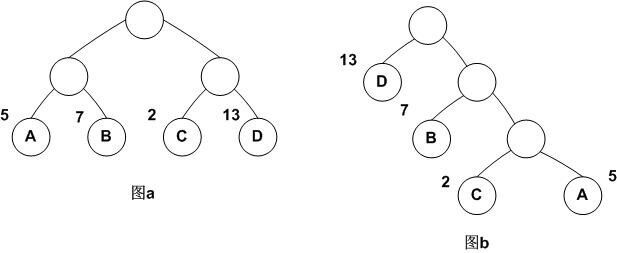左边是普通树，右边是哈夫曼树
图a： WPL=5*2+7*2+2*2+13*2=54
图b： WPL=5*3+2*3+7*2+13*1=48
可见，图b的带权路径长度较小，我们可以证明图b就是哈夫曼树(也称为最优二叉树)。

二. 怎么生成和计算？
1. 总结
①先对权值从小到大排序。
②选两个最小的加起来成为一个新结点，而这两个最小的值是新结点的左右子结点。
③两个老的结点去掉，新的结点放入再次排序然后重复过程②。
④直到完全生成一棵树。
⑤计算的时候，只计算那些初始权值里面有的值，把它乘以深度（和传统说的深度不一样，是传统说的深度减一）加起来就是路径长度。
2. 例子
例：对于给定的一组权值w={1,4,9,16,25,36,49,64,81,100},构造具有最小带权外部路径长度的扩充二叉树，并求出他的的带权外部路径长度。
解答过程（红色表示原来的权值结点，蓝色是加出来的结点）：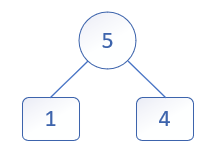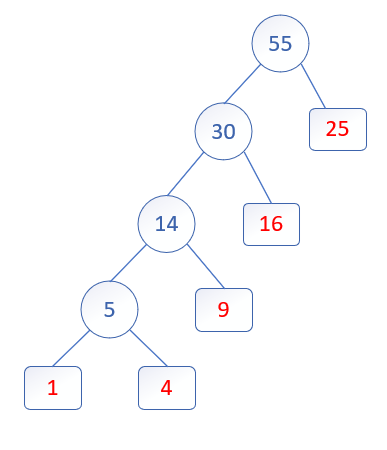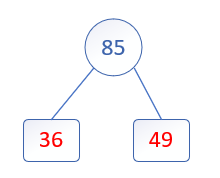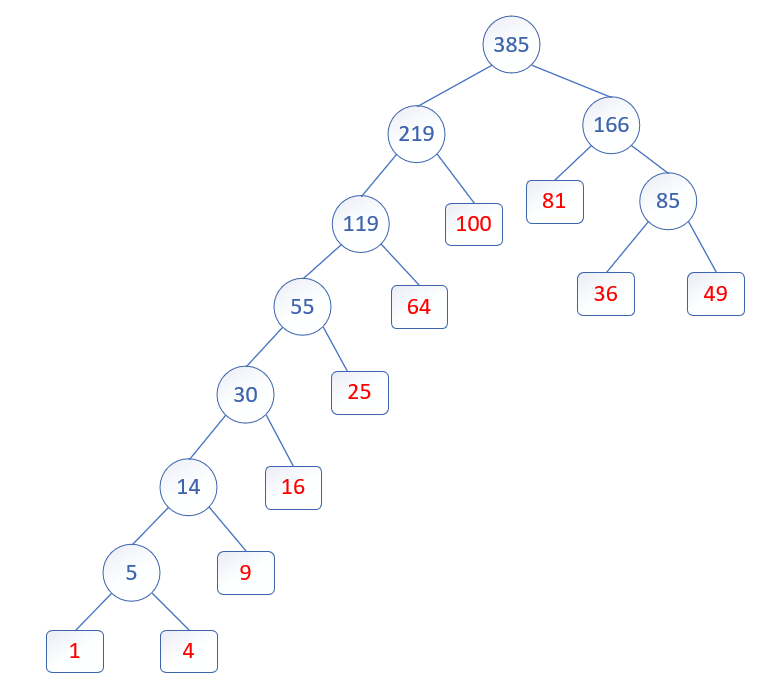带权外部路径长度计算：
WPL=2*100 + 3*64 + 2*81 + 4*25 + 3*49 + 3*36 + 5*16 + 6*9 + 7*1 + 7*4 =1078
展开全文• #include<stdio.h> #include<queue> using namespace std; priority_queue<int, vector<int> ,greater<int>> q; int main() { int n; while (scanf("%d", &... whi...
#include<stdio.h>
#include<queue>
using namespace std;
priority_queue<int, vector<int> ,greater<int>> q;
int main()
{
int n;
while (scanf("%d", &n) != EOF)
{
while (!q.empty())
q.pop();
for (int i = 0; i < n; i++)
{
int x;
scanf("%d", &x);
q.push(x);
}
int ans = 0;
while (q.size() > 1)
{
int a = q.top();
q.pop();
int b = q.top();
q.pop();
ans += a + b;
q.push(a+b);
}
printf("%d\n", ans);
}
return 0;
}

展开全文• 需要用这些叶结点生成哈夫曼树，根据哈夫曼树的概念，这些结点有权值，即weight，题目需要输出哈夫曼树带权路径长度（WPL）。 输入格式: 第一行输入一个数n，第二行输入n个叶结点（叶结点权值不超过1000，2<=n&...
题目描述： 哈夫曼树，第一行输入一个数n，表示叶结点的个数。 需要用这些叶结点生成哈夫曼树，根据哈夫曼树的概念，这些结点有权值，即weight，题目需要输出哈夫曼树的带权路径长度（WPL）。
输入格式: 第一行输入一个数n，第二行输入n个叶结点（叶结点权值不超过1000，2<=n<=1000）。
输出格式: 在一行中输出WPL值。
输入样例: 5 1 2 2 5 9
输出样例: 37
参考代码：
#include <stdio.h>

#define INF 65535

struct huffman{
int w;
int parent,lchild,rchild;
}HT;

int n;

void createHT()
{

int i,j;
//初始化
for(i=0;i<2*n-1;i++)
HT[i].parent=HT[i].lchild=HT[i].rchild=-1;

for(i=0;i<n;i++)
scanf("%d",&HT[i].w);

/*选用两小添新树
删除两小添新人。*/
int a,b;
int a1,b1;
for(i=0;i<n-1;i++)
{
a1=b1=INF;
for(j=0;j<n+i;j++)
{
if(HT[j].parent==-1&&HT[j].w<a1)
{
b=a;
a=j;
b1=a1;
a1=HT[j].w;
}
else if(HT[j].parent==-1&&HT[j].w<b1)
{
b=j;
b1=HT[j].w;
}
}

HT[n+i].w=HT[a].w+HT[b].w;
HT[n+i].lchild=a;
HT[n+i].rchild=b;
HT[a].parent=HT[b].parent=n+i;
}
}

int main()
{
while(scanf("%d",&n)!=EOF)
{
int i,sum=0;

createHT();
for(i=n;i<2*n-1;i++)
sum+=HT[i].w;

printf("%d\n",sum);
}
return 0;
}

参考资料： 传送门
展开全文算法 数据结构
• 此题的一种哈夫曼树形式如下（构造过程见另一篇博客） （从第0层开始）WPL = 2 * 3（长度为3的编码形式）+3*3 + （4+5+6）*2 = 45； 这是定义的计算WPL的方式，然后我们看一下另一个奇妙的结果20 + 9 + 11...


1002. Huffman coding

Time Limit: 1sec    Memory Limit:256MB

Description

In computer science and information theory, a Huffman code is an optimal prefix code algorithm.
In this exercise, please use Huffman coding to encode a given data.
You should output the number of bits, denoted asB(T), to encode the data:
B(T)=∑f(c)dT(c),
wheref(c) is the frequency of character c, and dT(c) is be the depth of characterc's leaf in the tree T.

Input

The first line is the number of characters n.
The following n lines, each line gives the character c and its frequencyf(c).

Output

Output a single number B(T).

Sample InputCopy sample input to clipboard

5
0 5
1 4
2 6
3 2
4 3

Sample Output

45

Hint

0: 01

1: 00

2: 11

3: 100

4: 101

题目的意思大致是（直接看测试样例）第一行为测试样例数，接下来n行，第一个为字符（不一定是整数的），第二个是字符出现的频率。
此题的一种哈夫曼树形式如下（构造过程见另一篇博客）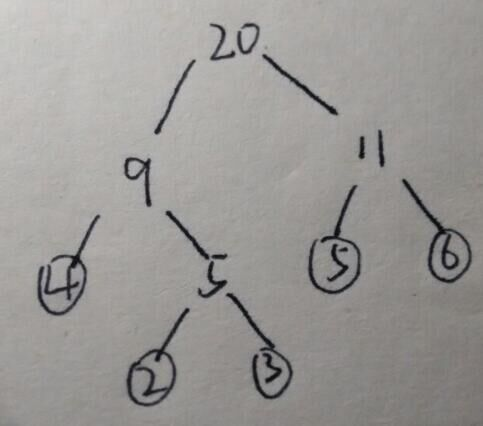（从第0层开始）WPL = 2 * 3（长度为3的编码形式）+3*3 + （4+5+6）*2 = 45；
这是定义的计算WPL的方式，然后我们看一下另一个奇妙的结果20 + 9 + 11 + 5 = 45；

证明如下：（证明并不充分
详细点的证明和讲解）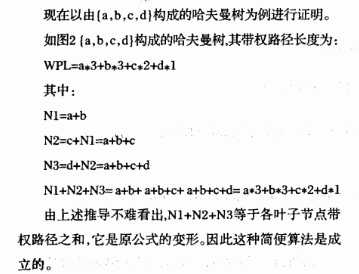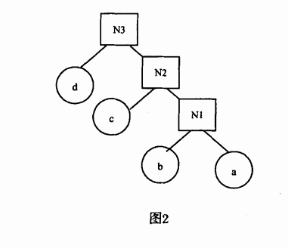如果知道了这个结论，这种题目的难度完全降没了。接下来就贴代码了。代码太渣不过还是拿出来 吧

#include<iostream>
#include<algorithm>

using namespace std;

int main()
{
int N;
cin >> N;
int hafuman[2*N];//some complier don't support.sometimes N should be const.
for(int i = 0;i < N;i++)
{
char index;
int fre;
cin >> index >> fre;
hafuman[i] = fre;
}
int ans = 0;
for(int i = 0;i < N-1;i++)
{
sort(hafuman+i,hafuman+N);
//		for(int j = i;j < N;j++) cout << hafuman[j] << " " ;
//		cout << endl;
//			cout << ans << " ans " << endl;
hafuman[i+1] = hafuman[i] + hafuman[i+1];
ans += hafuman[i+1];
}
cout << ans << endl;
}

另外还可以用优先队列（用小根堆的），这样就只需要每次比较对首两个元素。


展开全文• 哈夫曼树：一类带权路径最短的树。用于通讯及数据传送中构造传输效率最高的二进制编码（哈夫曼...树的路径长度：从树的根到树中每一个节点的路径长度之和； 下面以【5,7，10,12,18,20,28】为例（美团笔试题）： ...
• 一般的，我们是可以用常规的构造哈夫曼树带权路径长度。 计算结点的带权路径长度：结点到树根之间的路径长度与该结点上权的乘积。 带权路径长度WPL（Weighted Path Length）最小的二叉树，也称为最优二又树。 在...数据结构 算法
• 树的带权路径长度WPL 哈夫曼树构造 哈夫曼树性质 哈夫曼编码 试题数据结构
•  已知输入两行正整数，第二行正整数之间用空格键分开，请建立一个哈夫曼树，以输入的数字为叶节点，求这棵哈夫曼树带权路径长度。 【输入形式】  首先第一行为输入正整数的个数，然后接下来的一行正整数，代表叶...
• 哈夫曼树带权路径长度和：所有构造得到的中间结点（非叶子结点）的权值 构造中，每次寻找权值最小的两个结点，使用堆优化指logn #include<iostream> #include<cstdio> #include<queue&...
• 从根结点到叶子结点的路径长度乘以叶子节点权值，得到的值为该节点的带权路径长度，中所有叶子节点的带权路径长度之和称为该的带权路径长度和。给定N个结点和它们的权值，以这N个结点为叶子节点构造的带权路径...C语言
• 已知输入一串正整数，正整数之间用空格键分开，请建立一个哈夫曼树，以输入的数字为叶节点，求这棵哈夫曼树带权路径长度。 【输入形式】 首先输入正整数的个数，然后接下来为接下来的正整数，正整数个数不超过10个...
• 哈夫曼树带权路径长度 哈夫曼树是带权路径最小的一种特殊二叉树，所以也称最优二叉树。 在这里不讨论基本概念如如何计算路径等，而只着重于树的创建，具体过程让我们举例而言。 其基本的原理为：将所有节点一开始...
• ## 哈夫曼树带权路径

千次阅读 2018-06-05 18:19:53
Description:第一行有一个正整数n，代表单词的个数第二行...Sample Input:41 2 3 4Sample Output:19Hint:将第一个单词编码为000第二个单词编码为001第三个单词编码为01第四个单词编码为1总长度为3 * 1 + 3 * 2 + 2 *...
• 已知输入一串正整数，正整数之间用空格键分开，请建立一个哈夫曼树，以输入的数字为叶节点，求这棵哈夫曼树带权路径长度。 【输入形式】 首先输入正整数的个数，然后接下来为接下来的正整数，正整数个数不超过10个...python
• 树的带权路径长度(Weighted Path Length of Tree)：定义为树中所有叶结点的带权路径长度之和。 结点的带权路径长度：结点到树根之间的路径长度与该结点上权的乘积。 哈夫曼树是一种带权路径长度最短的二叉树，也...
•算法 队列 数据结构
• 在准备软考的过程中遇到哈夫曼树题型，有些...设二叉树具有N个带权值的叶子结点，从根结点到各个叶子结点的路径长度与相应叶子结点权值的乘积之和叫做二叉树的带权路径长度：WPL=w1l1+w2l2+…+wklk 其中wk为第K个...
• 哈夫曼树，又称最优二叉树，是一类带权路径长度最短的树。 构建哈夫曼树的算法如下： 对给定的n个权值{W1,W2,W3,...Wi,...,Wn}构成n个二叉树的初始集合F={T1,T2,T3,...Ti,...Tn},其中每棵二叉树Ti中只有一个权值为...
• ## 哈夫曼树结构和带权路径长度计算

万次阅读 多人点赞 2017-11-08 13:55:38
哈夫曼树是一种带权路径长度最短的二叉树，也称为最优二叉树。下面用一幅图来说明。 它们的带权路径长度分别为： 图a： WPL=5*2+7*2+2*2+13*2=54 图b： WPL=5*3+2*3+7*2+13*1=48 可见，...
• 假设我们一个权重为1,7,3,13,12,15,24怎么样画出哈夫曼树和计算带权路径长度。 首先，选出最小的两个权重值，这里是1,3（矩形表示叶子节点，圆表示根节点也是两个叶子节点的）如图： 然后，选出第三小的7，算...
• 需要用这些叶结点生成哈夫曼树，根据哈夫曼树的概念，这些结点有权值，即weight，题目需要输出哈夫曼树带权路径长度（WPL）。 输入格式: 第一行输入一个数n，第二行输入n个叶结点（叶结点权值不超过1000，2<=n&...优先级队列
• C语言自动计算哈夫曼树带权路径长度： #include<stdio.h> #include<stdlib.h> int cmp(const void *a,const void *b) { return *(int*)a-*(int *)b; } int main() { int n,W; while(scanf("%...算法
• #include #include using namespace std; typedef long long ll; int main() {  int n;  while(cin>>n)  {  priority_queue, greater >q;  for(int i=1; i  { ll t;
• 哈夫曼树是WPL（带权路径长度）最小的二叉树。使用这种思想，提出哈夫曼编码，节省空间。哈夫曼编码 最优二叉树
•  已知输入一串正整数，正整数之间用空格键分开，请建立一个哈夫曼树，以输入的数字为叶节点，求这棵哈夫曼树带权路径长度。 【输入形式】  首先输入正整数的个数，然后接下来为接下来的正整数，正整数个数不超过......